How to use Index Match function in multiple criteria

In this article we will learn how to retrieve an item from a list that meets multiple criteria, we use Index and Match function in Microsoft Excel.

MATCH: The Match formula returns the cell number where the value is found in a horizontal or vertical range.

Syntax of “MATCH” function:=MATCH(lookup_value,lookup_array,[match_type])

There is 3 match type 1st is 1-less than, 2nd is 0-Exact match, 3rd is (-1)-greater than. For example:- We have data in range A1: C5. Column A contains Order Id, column B contains Unit Price, and column C contains Quantity.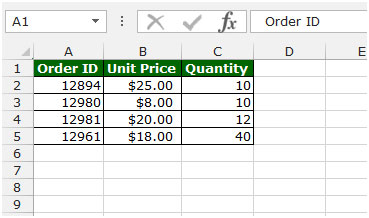Follow below given steps:-

• Write the formula in cell D2.
• =MATCH(12982,A2:A5,1), press Enter on your keyboard.
• The function will return 4. It means 4thcell is matching as per given criteria.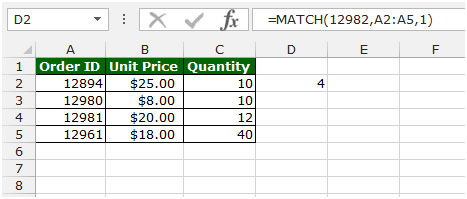INDEX: The Index formula returns a value from the intersection between the row number and the column number in an Array. There are 2 syntaxes for the “INDEX” function.

1stSyntaxof“INDEX”function: =INDEX (array, row_num, [column_num])

2nd Syntax of “INDEX” function:=INDEX (reference, row_num, [column_num], [area_num])

For example: - We have data in range A1:C5. Column A contains Order Id, column B contains Unit Price, and column C contains Quantity. Follow below given steps:-

• Write the formula in cell D2.
• =INDEX(A1:C5,2,3) press Enter on your keyboard.
• The function will return 10. It means 10 is available in 2nd row and 3rd column.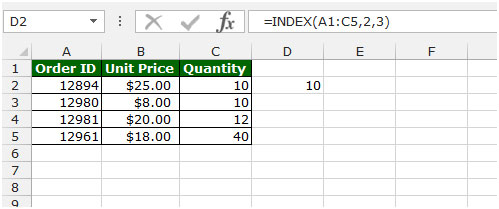Let’s take an example to understand how to retrieve an item from a list that meets multiple criteria. We have 2 data sets. Range 1 (A2:C7) contains file names with their matching types and sizes. Range 2 (A10:B15) contains a similar list of file names but with matching types only.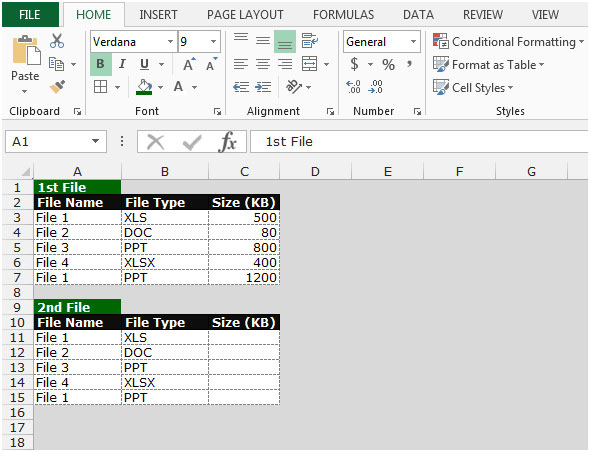Now, we want to find the appropriate file sizes from Range1 by matching each pair of file names and types from both the ranges. Follow below given steps:-

• Write the formula in cell C11.
• =INDEX(\$C\$3:\$C\$7,MATCH(A11&B11,\$A\$3:\$A\$7&\$B\$3:\$B\$7,0))
• Press Ctrl+Shift+Enter on your keyboard to create an array formula.
• {=INDEX(\$C\$3:\$C\$7,MATCH(A11&B11,\$A\$3:\$A\$7&\$B\$3:\$B\$7,0))}
• The function will return the file size after checking the multiple criteria.
• Copy the same formula and paste one by one in each cell.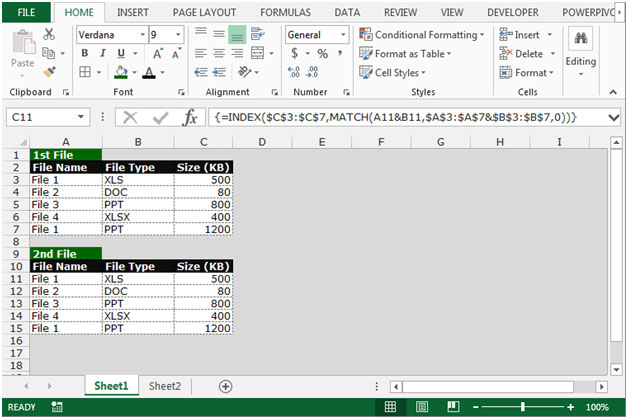This is the way we can retrieve the item from a list that meets multiple criteria by using the Index function along with Match function in Microsoft Excel.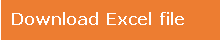1.